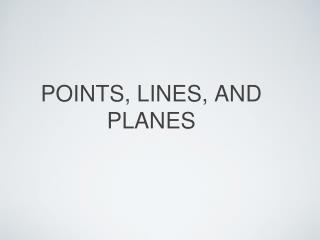DownloadDownload PresentationPOINTS, LINES, AND PLANES

# POINTS, LINES, AND PLANES

Télécharger la présentation## POINTS, LINES, AND PLANES

- - - - - - - - - - - - - - - - - - - - - - - - - - - E N D - - - - - - - - - - - - - - - - - - - - - - - - - - -
##### Presentation Transcript

1. POINTS, LINES, AND PLANES

2. UNDEFINED TERMS • Terms defined only by examples and descriptions • Point: a location • Line: a set of points that goes on infinitely in both directions and has no thickness or width • Plane: a flat surface made up of points

3. OTHER VOCABULARY • Collinear: on the same line • Coplanar: in the same plane • Space: a boundless three-dimensional set of all points • Locus: a set of points that satisfies a particular condition.

4. Name a line containing point A. • Name a plane containing point C.

5. How many planes are shaded in the figure? • Name three points that are collinear • Are points A, C, D, and J Coplanar? Explain.

6. PRACTICE • Pg. 9 #13-17, 21-35

7. Linear Measure and Precision

8. Precision: a measurement precise to within .5 units of measure Definitions • Line Segment: The part of a line between two end points • Between: point M is between points P and Q only if PM + MQ = PQ • Congruent: exactly the same size and shape

9. Find the precision for each measurement: Examples A. 5 millimeters Since the measurement is accurate to the nearest millimeter, the measurement must be accurate to the nearest .5 millimeter. So the precision is from 4.5 to 5.5 millimeters. B. 8 1/2 inches Since the measurement is accurate to the nearest 1/2 inch, the measurement must be accurate to the nearest 1/4 inch. So the precision is from 8 1/4 inches to 8 3/4 inches.

10. Examples

11. Practice • Pg. 17 #12-27, 34-39

12. Distance and Midpoints

13. Definitions • Midpoint-the point halfway between two points • Bisect-to cut in half

14. Midpoint Formula Average the x's and average the y's

15. Examples • Find the midpoint between (2,6) and (0,0) • Find the midpoint between (-5,-3) and (7,9)

16. Distance Formula

17. Examples • Find the distance between the points (2,5) and (5,9)

18. Examples • Find the distance between the points (0,5) and (5,-7)

19. Practice • Pg. 25 #13-22

20. ANGLE MEASURE

21. DEFINITIONS • Ray: a part of a line from one endpoint extending infinitely in one direction. • Angle: two rays that share an endpoint. • Vertex: the common endpoint of the two rays in an angle.

22. DEFINITIONS • Interior: inside of the angle. • Exterior: outside of the angle.

23. ANGLE CLASSIFICATIONS • Right angle: 1/4 of a circle; 90 degrees • Obtuse : greater than 90 degrees • Acute : less than 90 degrees

24. EXAMPLES • Name the vertex of angle 2 • C • Name the sides of angle 4 • BA and BC • What is another name for angle BDC • Angle CDB or Angle 1

25. EXAMPLES Angle RQT=47

26. EXAMPLES Angle TQS=22

27. PRACTICE • Pg. 34 #12-23

28. ANGLE RELATIONSHIPS

29. DEFINITIONS • Adjacent Angles: Angles that share a side and do not overlap: • Vertical Angles: two nonadjacent angles formed by two intersecting lines:

30. DEFINITIONS • Linear pair: a pair of adjacent angles that form a straight line: • Complementary Angles: a pair of angles whose sum is 90 degrees • Supplementary Angles: a pair of angles whose sum is 180 degrees • Perpendicular: lines that form right angles.

31. EXAMPLES Name a pair of adjacent angles One example: VZX AND XZW Name a pair of vertical angles VZX and YZW or VZY and XZW Name a linear pair Two examples: VZX and YZW or VZY and WZW

32. EXAMPLES

33. PRACTICE • Pg 42 #11-35

34. POLYGONS

35. DEFINITIONS • Not Polygons: • Polygons: • Polygon: a closed figure where all sides are segments • Regular Polygon: a polygon where all sides and all angles are congruent

36. DEFINITIONS • Concave and Convex: • Concave: • Convex: • Trick to remember: Concave figures have "hiding spots," just like a cave. • Perimeter: the sum of the lengths of the sides of a polygon.

37. NAMES OF POLYGONS

38. EXAMPLE

39. EXAMPLE

40. EXAMPLE WORK THROUGH ON BOARD 16 UNITS

41. PRACTICE • Pg. 48 #12-31